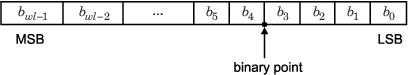定点数• bi 是第 i 个二进制数字。

• wl 是以位为单位的字长。

• bwl-1 是最高有效位或最高位 (MSB) 的位置。

• b0 是最低有效位或最低位 (LSB) 的位置。

• 二进制小数点显示在 LSB 左侧的四个位置处。因此，在此示例中，该数字称为有四个小数位，或者小数长度为 4。

二进制小数点的解释

Fixed-Point Designer 支持常规二进制小数点定标 V=Q*2^EV 是真实世界的值，Q 是存储的整数值，E 等于 -FractionLength。换言之，RealWorldValue = StoredInteger * 2 ^ -FractionLength

FractionLength 定义存储的整数值的定标。字长限制存储的整数可取的值，但不限制 FractionLength 可取的值。软件不会根据存储的整数 Q 的字长限制指数 E 的值。由于 E 等于 -FractionLength，因此没有必要将二进制小数点限制为要紧连着小数；小数长度可以是负数或大于字长。

$\begin{array}{l}bbb.=bbb.×{2}^{0}\\ bb.b=bbb.×{2}^{-1}\\ b.bb=bbb.×{2}^{-2}\\ .bbb=bbb.×{2}^{-3}\end{array}$

$\begin{array}{c}bbb00000.=bbb.×{2}^{5}\\ bbb00.=bbb.×{2}^{2}\\ .00bbb=bbb.×{2}^{-5}\\ .00000bbb=bbb.×{2}^{-8}\end{array}$

RealWorldValue = StoredInteger * 2 ^ -FractionLength。在本例中，为 RealWorldValue = 5 * 2 ^ -10 = 0.0048828125。由于小数长度比字长要长 2 位，因此存储的整数的二进制值是 x.xx00000101，其中 x 是隐式零的占位符。0.0000000101（二进制）等效于 0.0048828125（十进制）。有关使用 fi 对象的示例，请参阅 Fraction Length Greater Than Word Length (Fixed-Point Designer)